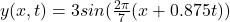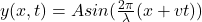## A water wave is 3m tall. Taking a picture of the wave, you measure 7m between peaks. With your stopwatch, you measure .8 seconds between a

Question

A water wave is 3m tall. Taking a picture of the wave, you measure 7m between peaks. With your stopwatch, you measure .8 seconds between a peak and a trough. Write the wave function for this water wave. Ignore the initial phi.

in progress 0
2 weeks 2021-08-30T19:25:11+00:00 1 Answers 0 views 0

The water wave function is given asExplanation:

Water waves are surface waves, which is a mixture of longitudinal and transverse waves.

The general wave equation is given as;where;

A is the amplitude = 3 m

λ is one wavelength = 7 m

t is period for one complete oscillation, T = 8 s

v is the velocity of the wave;

for each period, the wave  travels one wavelength, v = λ/T = 7/8 = 0.875m/sTherefore, the water wave function is given as